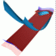Abstract and Contents
Next: 3.1 Iterating a fundamental domain
Previous: 2. Overview of earlier methods

# 3. The algorithm

We explain the algorithm for computing a two-dimensional unstable manifold of a normally hyperbolic invariant circle of saddle-type. How this algorithm can be used for the case of a hyperbolic fixed point is shown in Section 3.3. We begin by introducing some notation.

Let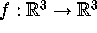be a diffeomorphism with a normally hyperbolic invariant circle H of saddle-type, and let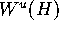be the unstable manifold of H. We assume that f can be transformed to a function defined on, where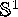is identified with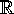, such that H is parametrized over. Then there exist a global linear foliation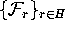that foliates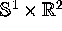.

The invariant circle H can be computed by the method in [Osinga 1996, Broer et al. 1996, Broer et al. 1997]. It is represented by a finite mesh M of points on H. The number of points in M can be prescribed. Suppose that the linear foliationsatisfies the Foliation Condition. (This is always true in a neighborhood of H; see also Section 6.) Recall that this means thatintersects each leaf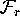in a unique curve. Our goal is to compute an approximation of the unique intersection curvefor the finitely many leaves of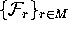.

To start the algortihm we need to know the first order approximation of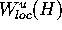in each leaf of. It is defined by unit vectorsthat are tangent to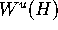. The vectors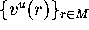can be obtained by interpolation from the embedded unstable normal bundlethat can be obtained from the Df-invariant splitting that is used in the method of [Osinga 1996, Broer et al. 1996, Broer et al. 1997]; see Figure 3. From now on we assume that the starting data for the algorithm, namely M,and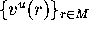, are known.

Next: 3.1 Iterating a fundamental domain
Previous: 2. Overview of earlier methodsAbstract and Contents

Written by: Bernd Krauskopf & Hinke Osinga
Created: May 27 1997 --- Last modified: Fri May 30 19:49:09 1997